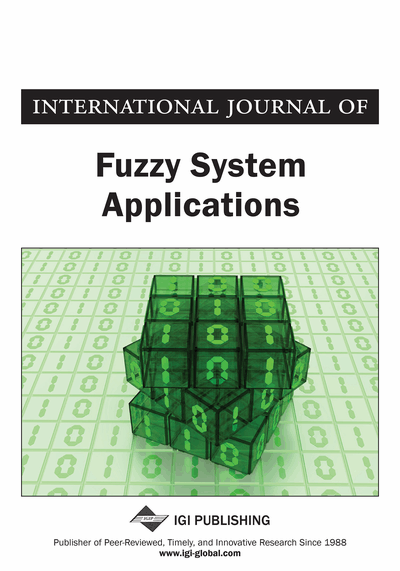# C̆ech Fuzzy Soft Closure Spaces

Rasha Naser Majeed (Mathematics Department, , College of Education for Pure Sciences Ibn Al- Haitham, Baghdad University, Baghdad, Iraq)
DOI: 10.4018/IJFSA.2018040103
Available
\$29.50
No Current Special Offers

## Abstract

In this paper, the C̆ech fuzzy soft closure spaces are defined and their basic properties are studied. Closed (respectively, open) fuzzy soft sets is defined in C̆ech fuzzy-soft closure spaces. It has been shown that for each C̆ech fuzzy soft closure space there is an associated fuzzy soft topological space. In addition, the concepts of a subspace and a sum are defined in C̆ech fuzzy soft closure space. Finally, fuzzy soft continuous (respectively, open and closed) mapping between C̆ech fuzzy soft closure spaces are introduced. Mathematics Subject Classification: 54A40, 54B05, 54C05.
Article Preview
Top

## 1. Introduction

The fundamental concept of a fuzzy set was introduced by Zadeh (1965) as a mathematical way to represent uncertainty and vagueness and to provide formalized tools for dealing with the imprecision intrinsic to many problems in everyday life. The notion of a fuzzy set has caused great interest among both `pure' and applied mathematicians. It has also raised enthusiasm among some engineers, biologists, psychologists, economists, and experts in other areas, who use (or at least try to use) mathematical ideas and methods in their research.

The discovery of fuzzy sets gave an incentive to many researchers to generalize the basic concepts of classical topology in fuzzy setting and thus a modern theory of fuzzy topology is developed. Chang (1968) introduced the notion of fuzzy topology when he replaced sets by fuzzy sets in the ordinary definition of topology. Since then, a lot of contributions to the development of fuzzy topology have been published (cf. (Lowen, 1976), (Mashhour et al., 1984), (Gähler, 1995), (Tantawy et al., 2015)). Parallel to this theory, there are also theories of probability, rough set theory which deal to solve these problems. Each of these theories has its potential difficulties as pointed out by Molodtsove (1999) who introduced the theory of soft sets, which is a new mathematical approch to solve the problems of an incomplete information.

Maji et al. (2001) introduced the concept of fuzzy soft sets by combining fuzzy sets and soft sets. The notion of fuzzy soft set is more generalized than that of fuzzy set and soft set. Later many researchers have applied the concept of fuzzy soft sets on different branches of mathematics, like group theory Aygünoğlu and Aygün (2009), Ghosh et al. (2011), decision making problems, relations Dinda and Samanta (2010), Ghosh and Samanta (2013), topology Tanay and Kandemir (2011), Roy and Samanta (2012), Roy and Samanta (2013), Roy and Samanta (2014).

The concept of C̆ech closure spaceswas introduced by C̆ech (1966), where C is a mapping from the power set of X to itself which satisfies the following conditions for all sets:,and. Mashhour and Ghanim (1985), introduced the concept of fuzzy closure spaces and C̆ech fuzzy closure spaces. Recently, Gowri and Jegadeesan (2014) used the soft set theory to define and study soft C̆ech closure spaces and separation axioms in soft C̆ech closure spaces. The soft closure operator in the sense of Gowri and Jegadeesan is defined from the soft power setto itself (where FA is a soft set over the universe set X with the set of parameter H, and. Another approach of C̆ech soft closure spaces is defined by Krishnaveni and Sekar (2014). In this approach, the soft closure operator is defined from the set of all soft sets over X to itself.

## Complete Article List

Search this Journal:
Reset
Volume 12: 1 Issue (2023)
Volume 11: 4 Issues (2022)
Volume 10: 4 Issues (2021)
Volume 9: 4 Issues (2020)
Volume 8: 4 Issues (2019)
Volume 7: 4 Issues (2018)
Volume 6: 4 Issues (2017)
Volume 5: 4 Issues (2016)
Volume 4: 4 Issues (2015)
Volume 3: 4 Issues (2013)
Volume 2: 4 Issues (2012)
Volume 1: 4 Issues (2011)
View Complete Journal Contents Listing